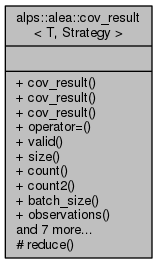ALPSCore reference
alps::alea::cov_result< T, Strategy > Class Template Reference

#include <covariance.hpp>

Collaboration diagram for alps::alea::cov_result< T, Strategy >:## Public Types

typedef bind< Strategy, T >::value_type value_type

typedef bind< Strategy, T >::var_type var_type

typedef bind< Strategy, T >::cov_type cov_type

## Public Member Functions

cov_result ()

cov_result (const cov_data< T, Strategy > &acc_data)

cov_result (const cov_result &other)

cov_resultoperator= (const cov_result &other)

bool valid () const

size_t size () const

size_t count () const

double count2 () const

double batch_size () const

double observations () const

const column< T > & mean () const

column< var_typevar () const

eigen< cov_type >::matrix cov () const

column< var_typestderror () const

const cov_data< T, Strategy > & store () const

cov_data< T, Strategy > & store ()

void reduce (const reducer &r)

## Protected Member Functions

void reduce (const reducer &, bool do_pre_commit, bool do_post_commit)

## Friends

class cov_acc< T, Strategy >

void serialize (serializer &, const std::string &, const cov_result &)

void deserialize (deserializer &, const std::string &, cov_result &)

std::ostream & operator<< (std::ostream &, const cov_result &)

## Detailed Description

### template<typename T, typename Strategy = circular_var> class alps::alea::cov_result< T, Strategy >

Accumulator which tracks the mean and a naive variance estimate.

Definition at line 22 of file covariance.hpp.

## Member Typedef Documentation

template<typename T, typename Strategy = circular_var>
 typedef bind::cov_type alps::alea::cov_result< T, Strategy >::cov_type

Definition at line 211 of file covariance.hpp.

template<typename T, typename Strategy = circular_var>
 typedef bind::value_type alps::alea::cov_result< T, Strategy >::value_type

Definition at line 209 of file covariance.hpp.

template<typename T, typename Strategy = circular_var>
 typedef bind::var_type alps::alea::cov_result< T, Strategy >::var_type

Definition at line 210 of file covariance.hpp.

## Constructor & Destructor Documentation

template<typename T, typename Strategy = circular_var>
 alps::alea::cov_result< T, Strategy >::cov_result ( )
inline

Definition at line 214 of file covariance.hpp.

template<typename T, typename Strategy = circular_var>
 alps::alea::cov_result< T, Strategy >::cov_result ( const cov_data< T, Strategy > & acc_data )
inline

Definition at line 216 of file covariance.hpp.

template<typename T , typename Str >
 alps::alea::cov_result< T, Str >::cov_result ( const cov_result< T, Strategy > & other )

Definition at line 207 of file covariance.cpp.

## Member Function Documentation

template<typename T, typename Strategy = circular_var>
 double alps::alea::cov_result< T, Strategy >::batch_size ( ) const
inline

Returns average batch size

Definition at line 237 of file covariance.hpp.

template<typename T, typename Strategy = circular_var>
 size_t alps::alea::cov_result< T, Strategy >::count ( ) const
inline

Returns sample size, i.e., number of accumulated data points

Definition at line 231 of file covariance.hpp.

template<typename T, typename Strategy = circular_var>
 double alps::alea::cov_result< T, Strategy >::count2 ( ) const
inline

Returns sum of squared sample sizes

Definition at line 234 of file covariance.hpp.

template<typename T, typename Strategy = circular_var>
 eigen::matrix alps::alea::cov_result< T, Strategy >::cov ( ) const
inline

Returns bias-corrected sample covariance matrix

Definition at line 253 of file covariance.hpp.

template<typename T, typename Strategy = circular_var>
 const column& alps::alea::cov_result< T, Strategy >::mean ( ) const
inline

Returns sample mean

Definition at line 243 of file covariance.hpp.

template<typename T, typename Strategy = circular_var>
 double alps::alea::cov_result< T, Strategy >::observations ( ) const
inline

Returns effective number of observations

Definition at line 240 of file covariance.hpp.

template<typename T , typename Str >
 cov_result< T, Str > & alps::alea::cov_result< T, Str >::operator= ( const cov_result< T, Strategy > & other )

Definition at line 212 of file covariance.cpp.

template<typename T, typename Strategy = circular_var>
 void alps::alea::cov_result< T, Strategy >::reduce ( const reducer & r )
inline

Collect measurements from different instances using sum-reducer

Definition at line 265 of file covariance.hpp.

template<typename T , typename Str >
 void alps::alea::cov_result< T, Str >::reduce ( const reducer & r, bool do_pre_commit, bool do_post_commit )
protected

Definition at line 244 of file covariance.cpp.

template<typename T, typename Strategy = circular_var>
 size_t alps::alea::cov_result< T, Strategy >::size ( ) const
inline

Number of components of the random vector (e.g., size of mean)

Definition at line 228 of file covariance.hpp.

template<typename T , typename Str >
 column< typename cov_result< T, Str >::var_type > alps::alea::cov_result< T, Str >::stderror ( ) const

Returns bias-corrected standard error of the mean

Definition at line 237 of file covariance.cpp.

template<typename T, typename Strategy = circular_var>
 const cov_data& alps::alea::cov_result< T, Strategy >::store ( ) const
inline

Return backend object used for storing estimands

Definition at line 259 of file covariance.hpp.

template<typename T, typename Strategy = circular_var>
 cov_data& alps::alea::cov_result< T, Strategy >::store ( )
inline

Return backend object used for storing estimands

Definition at line 262 of file covariance.hpp.

template<typename T, typename Strategy = circular_var>
 bool alps::alea::cov_result< T, Strategy >::valid ( ) const
inline

Returns false if finalize() has been called, true otherwise

Definition at line 225 of file covariance.hpp.

template<typename T, typename Strategy = circular_var>
 column alps::alea::cov_result< T, Strategy >::var ( ) const
inline

Returns bias-corrected sample variance

Definition at line 250 of file covariance.hpp.

## Friends And Related Function Documentation

template<typename T, typename Strategy = circular_var>
 friend class cov_acc< T, Strategy >
friend

Definition at line 282 of file covariance.hpp.

template<typename T, typename Strategy = circular_var>
 void deserialize ( deserializer & , const std::string & , cov_result< T, Strategy > & )
friend

Convert result from a permanent format (write to disk etc.)

template<typename T, typename Strategy = circular_var>
 std::ostream& operator<< ( std::ostream & , const cov_result< T, Strategy > & )
friend

Write some info about the result to a stream

template<typename T, typename Strategy = circular_var>
 void serialize ( serializer & , const std::string & , const cov_result< T, Strategy > & )
friend

Convert result to a permanent format (write to disk etc.)

The documentation for this class was generated from the following files: